### Choose language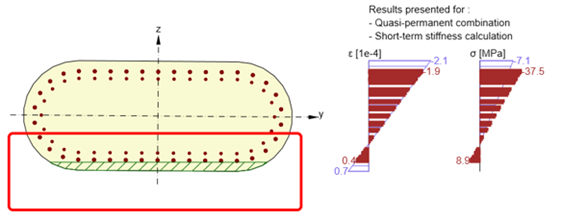# Crack width check of cross-sections with a large concrete cover

The crack width check is an important and integral part of the structural report. If you code-check reinforced concrete cross-sections with a large concrete cover, you may have got into trouble; specifically, the check cannot be performed.
$$For the calculation of the crack width according to EN 1992-1-1, chapter 7.3.4, the depth of effective tension area hc,eff and reinforcement ratio ρc,eff need to be evaluated. The depth hc,eff is determined according to this formula: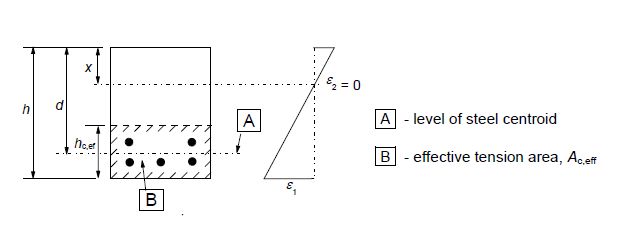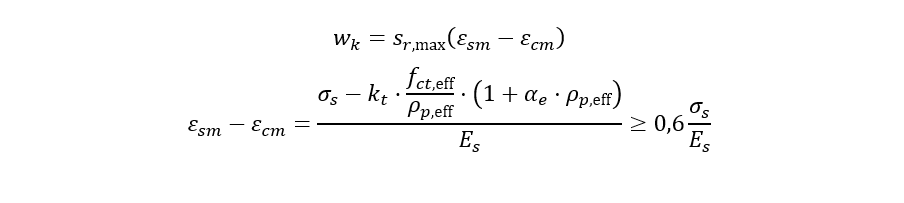There are cases where the cross-section is subjected to a bending moment and a compressive normal force, while at the same time a large reinforcement cover is designed. This situation occurs mainly in bridge substructures, piers etc. In this case, the depth of effective tension area leads to a value of zero due to a large concrete cover: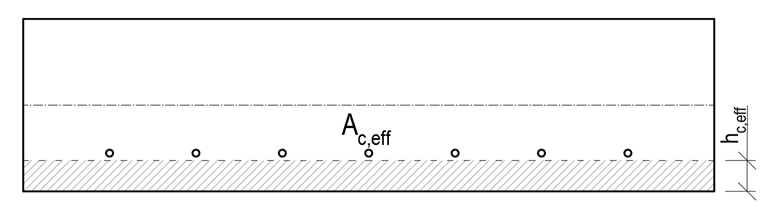The Eurocode methodology could not be applied since no reinforcement was found in the tension area. No reinforcement in the tension zone, no crack width check. But engineers need to have this check done. How did we deal with satisfying both engineer's needs and code requirements?Since several enhancements have been made to the crack width check over the last four releases, let's re-visit these particular enhancements and present the newest, of course.Version 20.0 and olderBy version 20.0, the absence of reinforcement in the Ac,eff region was resolved by using the right-hand side of the equation (7.3.4) to calculate the difference of the relative strains of concrete and reinforcement:where stress in the most tensioned reinforcement was substituted.And the maximum crack spacing was calculated according to Equation 7.14, i.e.: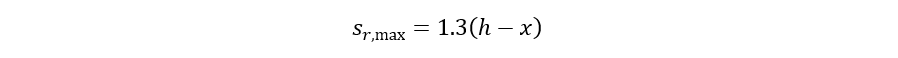If the issue with no reinforcement in the tension zone came to happen, the above-mentioned workaround was applied.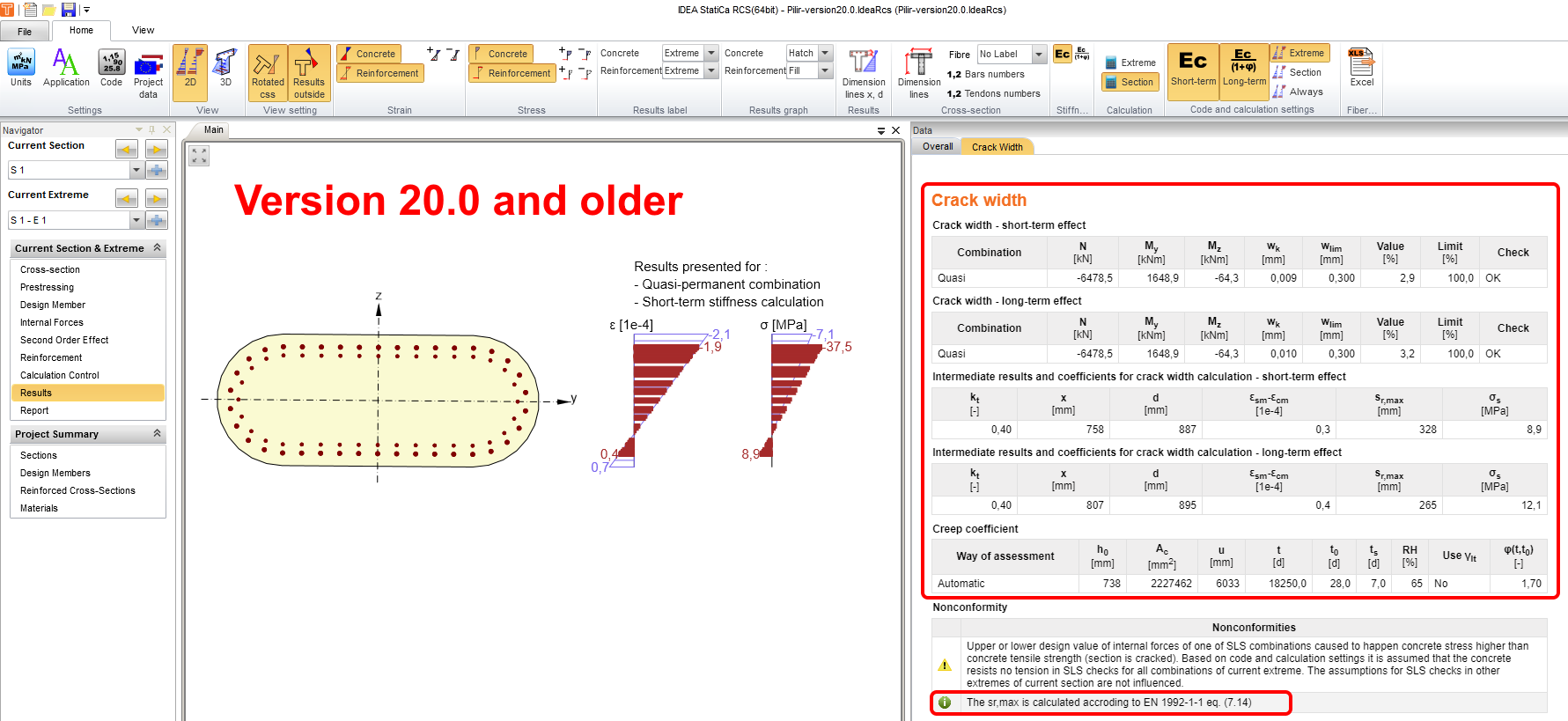Version 20.1Since the calculation used in Version 20.0 and older does not meet the basic assumptions of the tension chord model (reinforcing bar embedded in concrete), we decided to not use the workaround implemented in version 20.0 and older. And if the reinforcement was out of the tension zone, a user was warned by the nonconformity:No tensile reinforcement was found in the tension area of the cross-section. Therefore the tensile reinforcement cannot be determined and crack width cannot be calculated acc. to clause 7.3.4.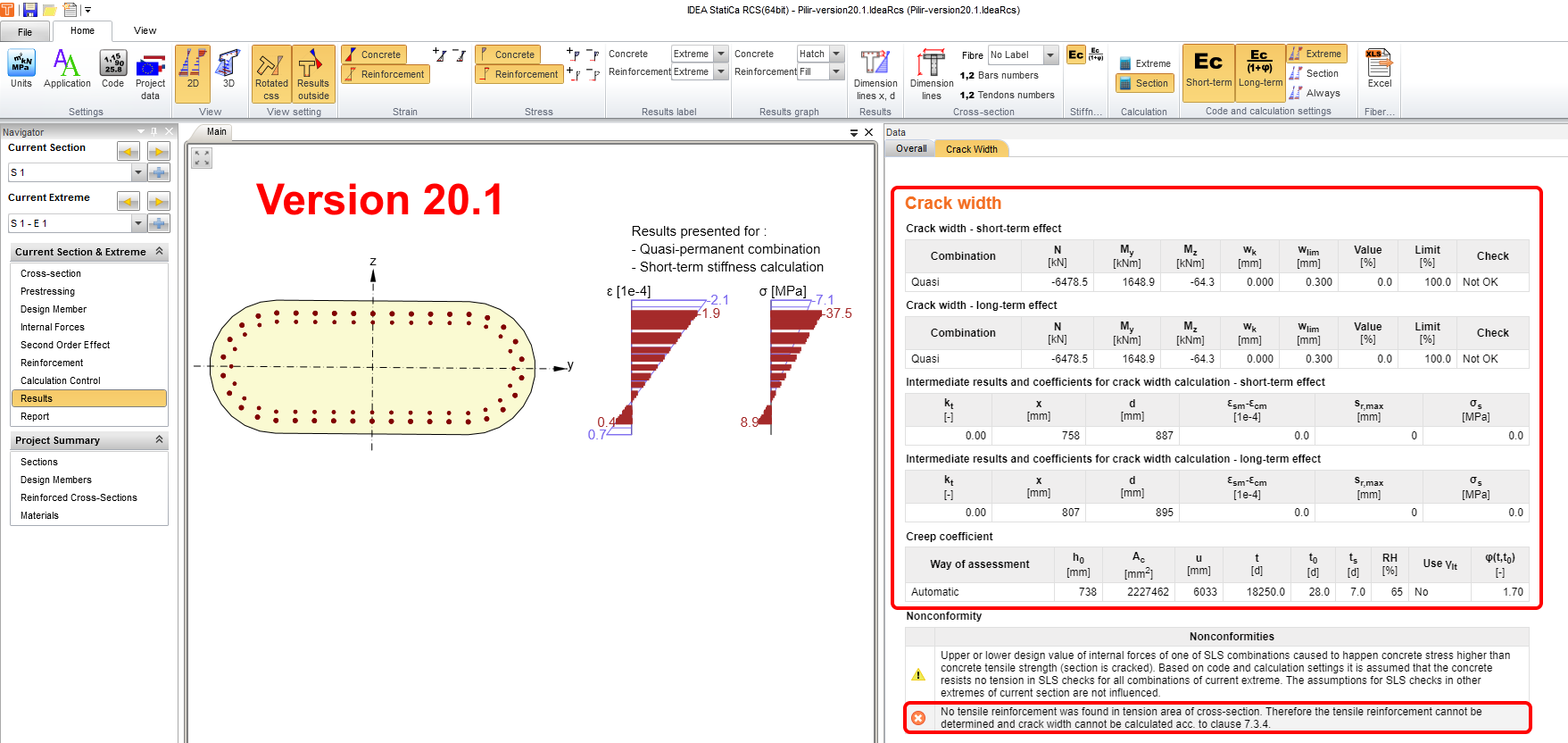Version 21.0The only change compared to version 20.1 is that we started to display all the values we are able to count, at the same time we started to display the effective tensile area of the concrete in the scene. This allows the user to see that all the reinforcement is outside the Ac,eff region. Note that the center of gravity of the reinforcement must be inside of the tension area to consider it as embedded reinforcement. At the same time, a nonconformity was displayed:No tensile reinforcement was found in the tension area of the cross-section. Therefore the tensile reinforcement cannot be determined and crack width cannot be calculated acc. to clause 7.3.4.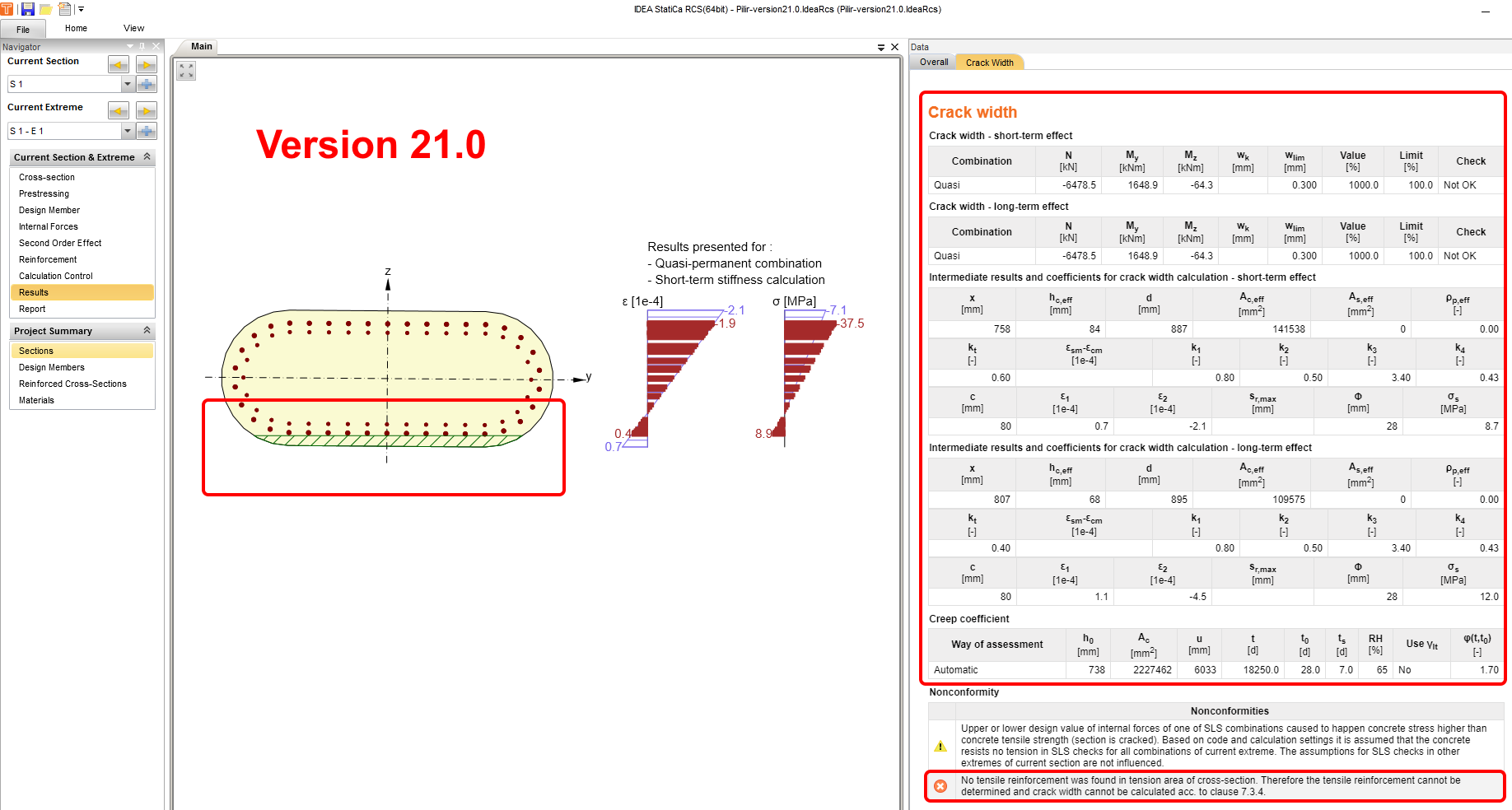Version 21.1When determining the effective tension area, for each case of crack width calculation, the calculation is performed as follows: Step 1 – a regular calculation is performed, where the height hc,ef is determined as: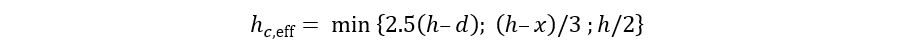Step 2 – if the effective depth hc,eff is so small that all the reinforcement lies outside this region, the depth is determined as described in step 3. Step 3 – hc,eff calculation excluding the critical condition is determined as: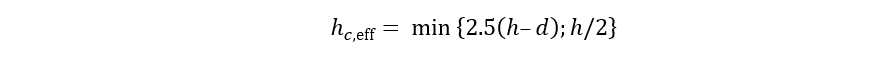At the same time, the following nonconformity is displayed:The effective area of concrete in tension surrounding the reinforcement or prestressing tendons of depth hc,eff, where hc,eff is the lesser of 2.5(h – d) or h/2. In the case of considering of value as (h – x)/3, the reinforcement is out of the effective area of concrete in tension, and therefore it would not be possible to calculate crack width according to clause 7.3.4.Note that this enhancement was already released in the patch of version 21.0, i.e. 21.0.4.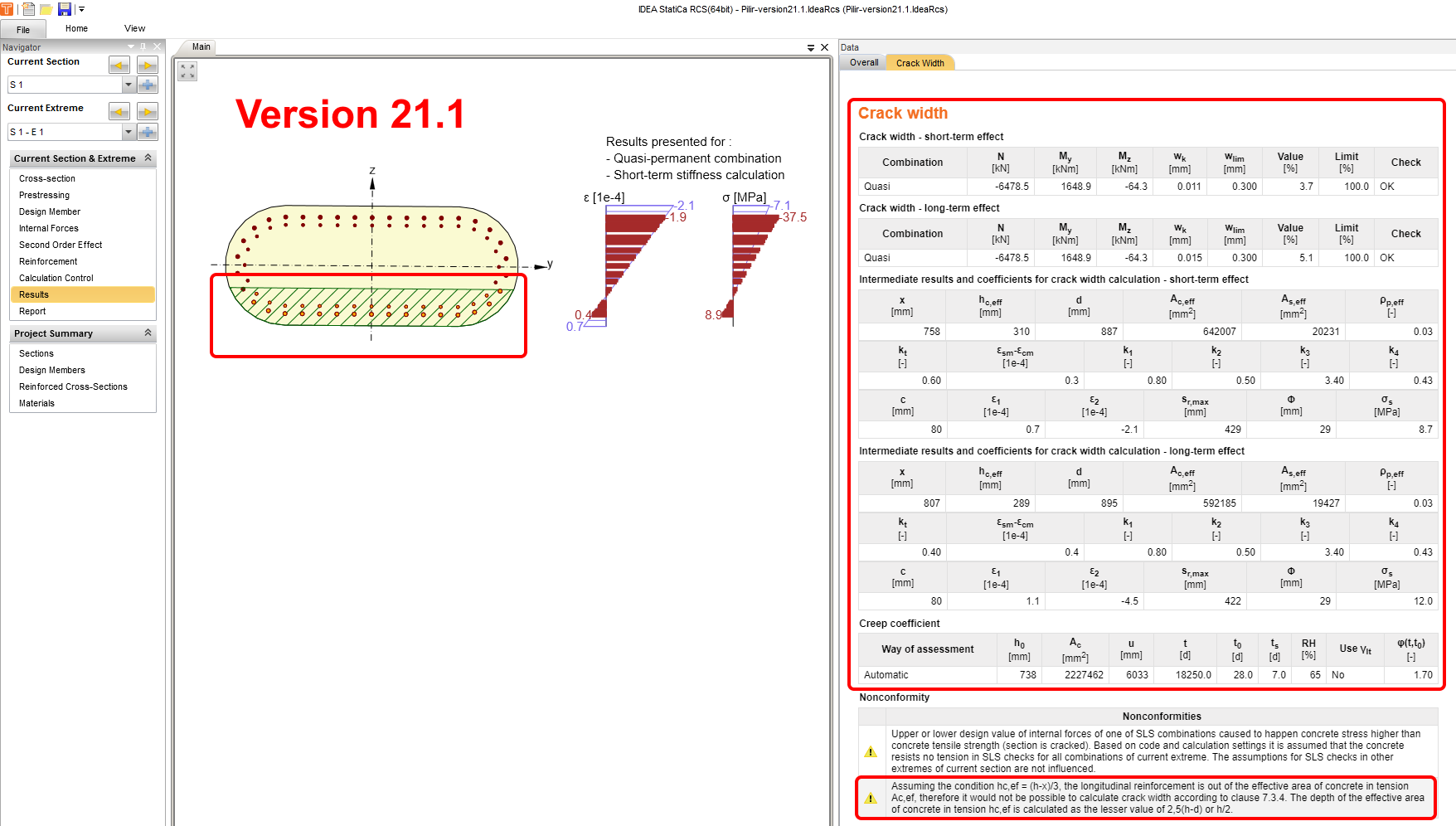This improvement will lead to more consistent results for crack calculations in IDEA StatiCa RCS, despite the limitations set in the code.Crack width check of reinforced concrete cross-sections with a large concrete cover is available in Concrete Expert, Concrete Enhanced, Prestressing Expert and Prestressing Enhanced edition of IDEA StatiCa.$$